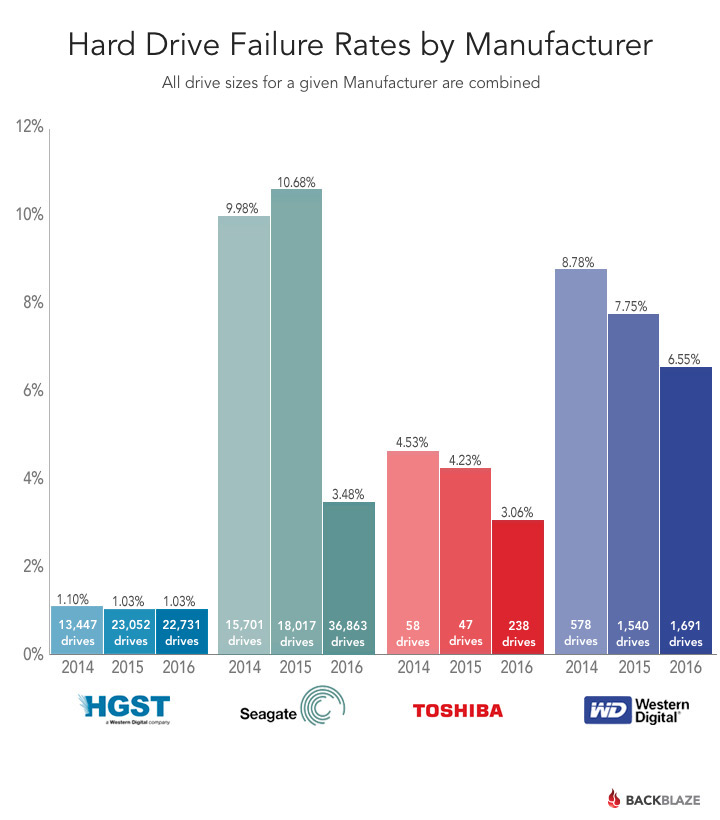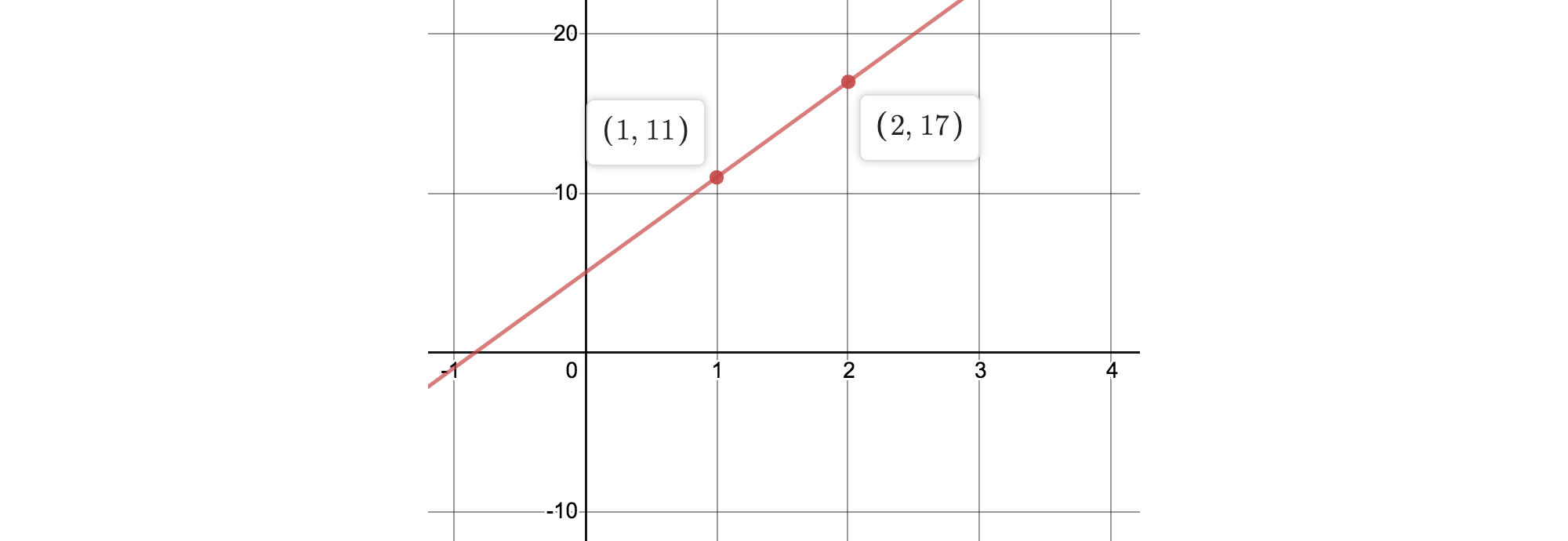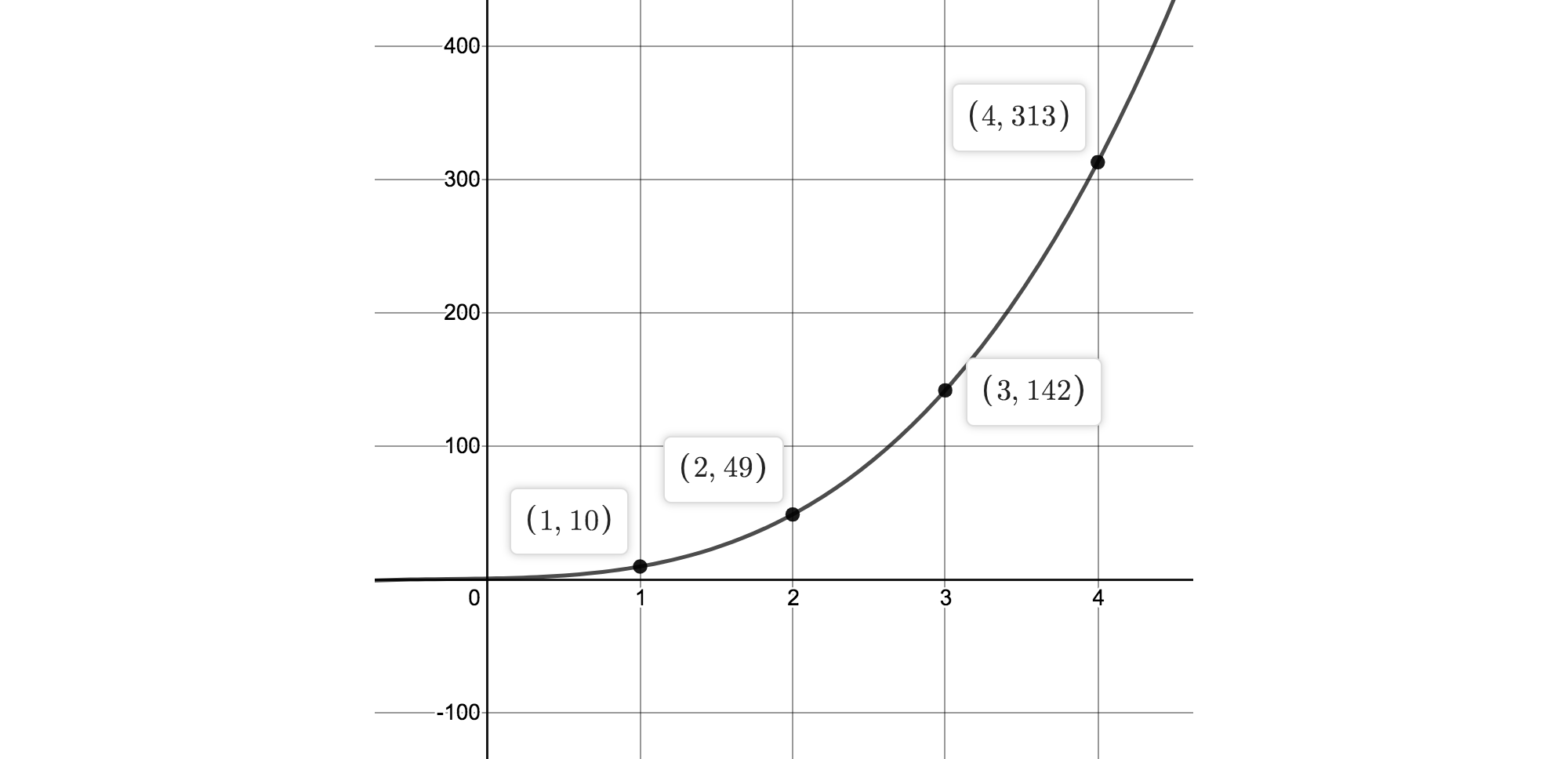# Erasure-Code-擦除码-1-原理篇

22 分钟阅读# 问题3副本的方式虽然简单容易实现, 但要额外浪费2倍的存储空间, 因此存储领域中一直都希望用一种较少的冗余的存储方式, 来实现同样较高的可靠性.

RAID 本质上跟EC没有区别, 它是单机系统时代被广泛使用的成熟实现. EC可以认为是分布式系统发展起来后, RAID算法在多机系统上的重新实现:

• RAID-0 相当于单副本;
• RAID-1 相当于2副本;
• RAID-5 相当于EC的k+1模式, k个数据块+1个校验块;
• RAID-6 相当于EC的k+2模式, k个数据块+2个校验块;

# EC的基本原理

EC的目标可以简单的理解为: 对k个同样大小的数据块, 额外增加m个校验块, 以使得这k+m个数据中任意丢失m个数据块/校验块时都能把丢失的数据找回.

## 栗子1: 实现k+1的冗余策略

🌰

Q: 有3个自然数, 能否做到再记录第4个数字, 让任何一个数字丢失的时候都可以将其找回?

• 存储过程:

就是存储这4个数字: d₁, d₂, d₃, y₁.

• 恢复过程:

• 如果 d₁, d₂, d₃ 任意一个丢失, 例如 d₁ 丢失了, 我们都可以通过 d₁ = y₁ - d₂ - d₃ 来得到 d₁ .

• 如果 y₁ 丢失, 则再次取 d₁ + d₂ + d₃ 的和就可以将 y₁ 找回.

2副本 200% 允许丢1块: 1 X 10⁻⁸ (d₁,d₁), (d₂,d₂), (d₃,d₃)
3+1求和冗余 133% 允许丢1块: 6 X 10⁻⁸ (d₁, d₂, d₃, y₁)

## 栗子2: 实现k+2的冗余策略

🌰🌰

Q: 有3块数据: d₁, d₂, d₃ 可否另外再存储2个冗余的校验块(共5块), 让整个系统任意丢失2份数据时都能找回?

k+1求和的策略里, 我们给数据块和校验块建立了一个方程, 把它们关联起来了: y₁ = d₁ + d₂ + d₃.

$\begin{cases} d_1 + d_2 + d_3 = y_1 \\ d_1 + d_2 + d_3 = y_2 \end{cases}$
• 存储过程:

存储 d₁, d₂, d₃, y₁, y₂ 这5个数字.

• 恢复过程:

如果 d₁, d₂ 都丢失了(用 u₁, u₂ 表示丢失的数据), 下面这个关于 u₁, u₂ 的线性方程是有无穷多解的:

$\begin{cases} u_1 + u_2 = y_1 - d_3 \\ u_1 + u_2 = y_2 - d_3 \end{cases}$

我们没有办法从这个方程组里解出 u₁, u₂ 的值, 因为第2个方程跟第1个一毛一样, 没有提供更多的信息.

• 计算 y₁ 时, 对每个数字乘以1, 1, 1, 1 …
• 计算 y₂ 时, 对每个数字乘以1, 2, 4, 8 …
\begin{aligned} y_1 & = d_1 + d_2 + d_3 \\ y_2 & = d_1 + 2 d_2 + 4 d_3 \end{aligned}

• 存储过程:

存储 d₁, d₂, d₃, y₁, y₂ 这5个数字.

• 数据恢复:

如果 d₁d₂ 之一丢失,恢复的过程跟k+1策略一样;

如果 d₁, d₂ 丢失(同样用 u₁, u₂ 表示), 我们可以使用剩下的3个数字 d₃, y₁, y₂ 来建里1个关于 u₁, u₂ 的二元一次方程组:

\begin{cases} \begin{aligned} u_1 + u_2 & = y_1 - d_3 \\ u_1 + 2 u_2 & = y_2 - 4 d_3 \end{aligned} \end{cases}

RAID-6为k个数据块(例如k=10)之外再多存储2个校验数据, 当整个系统丢失2块数据时, 都可以找回.

## 栗子3: 实现k+m的冗余策略

🌰🌰🌰

y₃ 的计算我们需要再为所有的 dⱼ 选择1组不同的系数, 例如1,3,9,27…来保证后面数据丢失时,得到的1个3元一次方程组是可解的:

\begin{cases} \begin{aligned} d_1 + d_2 + d_3 & = y_1 \\ d_1 + 2 d_2 + 4 d_3 & = y_2 \\ d_1 + 3 d_2 + 9 d_3 & = y_2 \end{aligned} \end{cases}

# EC的几何解释

## 2+m的冗余的本质: 两点确定一条直线

$y = d_1 + d_2 x$

• 这条直线包含的所有数据块 dⱼ 的信息:
• 任何1对 d₁, d₂ 的值就确定一条不同的直线.
• 同样, 任意1条直线也唯一对应1对 d₁, d₂ 的值.

• 我们要保存足够多的关于这条直线的信息, 能够在需要的时候找回这条直线. 然后再提取直线方程的系数来找回最初存储的数据块 d₁, d₂.• 存储过程:

d₁, d₂ 作为系数建立一个直线方程, 再在直线上取2个点, 记录点的坐标(这里我们总是取x = [1, 2, 3…]的自然数的值, 所以只记录y的值就可以了): d₁, d₂, (1, y₁), (2, y₂).

• 恢复过程:

已知过直线2点 (1, y₁), (2, y₂) 来确定直线方程, 再提取方程的系数.

$\begin{cases} y_1 = d_1 + d_2 \\ y_2 = d_1 + 2d_2 \end{cases}$

## k+m的冗余的本质: 高次曲线

$y = d_1 + d_2 x + d_3 x^2 + ... + d_k x^{k-1}$• 存储过程:

取m个不同的x的值(1, 2, 3…m), 记录这条曲线上m个不同点的坐标:

$(1, y₁), (2, y₂) ... (m, y_m)$

存储所有k个数据块 d₁, d₂ .... 和所有m个校验块 y₁, y₂ ....

• 恢复过程:

平面上m个点可以唯一确定1条 m-1 次幂的曲线(或通过m个点跟k-m个已知系数确定一条k-1次幂的曲线). 确定了这条关于x的曲线,就找回了它的系数,也就是数据块

$d_1, d_2 ... d_k$

## 从曲线方程到生成矩阵

\begin{aligned} y_1 = d_1 + 1 d_2 + 1^2 d_3 + \dots 1^{k-1} d_k \\ y_2 = d_1 + 2 d_2 + 2^2 d_3 + \dots 2^{k-1} d_k \\ y_3 = d_1 + 3 d_2 + 3^2 d_3 + \dots 3^{k-1} d_k \\ ... \end{aligned}

$\begin{bmatrix} y_1 \\ y_2 \\ y_3 \\ ... \\ y_m \end{bmatrix} = \begin{bmatrix} 1 & 1 & 1^2 & ... & 1^{k-1} \\ 1 & 2 & 2^2 & ... & 2^{k-1} \\ 1 & 3 & 3^2 & ... & 3^{k-1} \\ ... & ... & ... & ... & ... \\ 1 & m & m^2 & ... & m^{k-1} \end{bmatrix} \times \begin{bmatrix} d_1 \\ d_2 \\ d_3 \\ ... \\ d_k \end{bmatrix}$

Vandermonde 矩阵只是 EC 其中1种系数的选择方式. 其他常用的系数矩阵还有 Cauchy 矩阵等.

# EC的解码: 求解n元一次方程组

EC生成校验块的过程称之为EC的编码, 也就是用Vandermonde矩阵去乘所有的数据块.

$\begin{bmatrix} 1 & 1 & 1 & ... & 1 \\ 1 & 2 & 4 & ... & 2^{k-1} \\ 1 & 3 & 9 & ... & 3^{k-1} \\ ... \\ 1 & m & m^1 & ... & m^{k-1} \end{bmatrix} \times \begin{bmatrix} d_1 \\ d_2 \\ d_3 \\ ... \\ d_k \end{bmatrix} = \begin{bmatrix} y_1 \\ y_2 \\ y_3 \\ ... \\ y_m \end{bmatrix}$

$\begin{bmatrix} 1 & 1 & 1 & ... & 1 \\ 1 & 2 & 4 & ... & 2^{k-1} \\ \end{bmatrix} \times \begin{bmatrix} d_1 \\ u_2 \\ u_3 \\ ... \\ d_k \end{bmatrix} = \begin{bmatrix} y_1 \\ y_2 \\ \end{bmatrix}$

## Vandermonde 矩阵保证方程组有解

$V=\begin{bmatrix} 1 & x_1 & x_1^2 & \dots & x_1^{n-1} \\ 1 & x_2 & x_2^2 & \dots & x_2^{n-1} \\ 1 & x_3 & x_3^2 & \dots & x_3^{n-1} \\ \vdots & \vdots & \vdots & \ddots & \vdots \\ 1 & x_m & x_m^2 & \dots & x_m^{n-1} \end{bmatrix}$
• Vandermonde 的 行列式的值为:

$\det(V)=\prod_{1 \leq i \lt j \leq n}(x_j - x_i)$

只要 xᵢ 都不同, 则 Vandermonde 矩阵的行列式就不为0, 矩阵可逆, 表示方程有唯一解.

• Vandermonde 矩阵的任意 m x m 的子矩阵, 是一个Generalized Vandermonde Matrix, 它在 xᵢ 都为正数时可以保证永远有唯一解.

因此在实数领域, 我们总能保证上面这个冗余策略很好的工作.

EC擦除码系列: